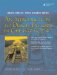# Functions with Variable-Length Argument Lists

In C and in C++ it is possible to define functions that have parameter lists ending with an ellipsis (. . .). The ellipsis allows the number of parameters and their types to be specified by the caller. The usual example of such a function is from .

```int printf(char* formatstr, ...)
```

This flexible mechanism permits calls such as

```printf("Eschew Obfuscation!
");
printf("%d days hath %s
", 30, "September");
```

To define a function that uses the ellipsis you need to

```#include
```

which adds to the std namespace a set of macros for accessing the items in the argument list. There must be at least one parameter other than the ellipsis in the parameter list. A variable, usually named ap (argument pointer), of type va_list is used to traverse the list of unnamed arguments. The macro

```va_start(ap, p)
```

where p is the last named parameter in the list, initializes ap so that it points to the first of the unnamed arguments. The macro

```va_arg(ap, typename)
```

returns the argument that ap is pointing to and uses the typename to determine (i.e., with sizeof) how large a step to take to find the next argument. The macro

```va_end(ap)
```

must be called after all of the unnamed arguments have been processed. It cleans up the unnamed argument stack and ensures that the program will behave properly after the function has terminated.

Example 24.1 shows how to use these features.

##### Example 24.1. src/ellipsis/ellipsis.cpp

 ```#include #include using namespace std; double mean(int n ...) { <-- 1 va_list ap; <-- 2 double sum(0); int count(n); va_start(ap, n); <-- 3 for(int i = 0; i < count; ++i) { sum += va_arg(ap, double); } va_end(ap); <-- 4 return sum / count; } int main() { cout << mean(4, 11.3, 22.5, 33.7, 44.9) << endl; cout << mean (5, 13.4, 22.5, 123.45, 421.33, 2525.353) << endl; } ``` (1)First parameter is number of args.(2)Sequentially points to each unnamed arg.(3)ap now points to first unnamed arg.(4)Clean up before returning.An Introduction to Design Patterns in C++ with Qt 4
ISBN: 0131879057
EAN: 2147483647
Year: 2004
Pages: 268